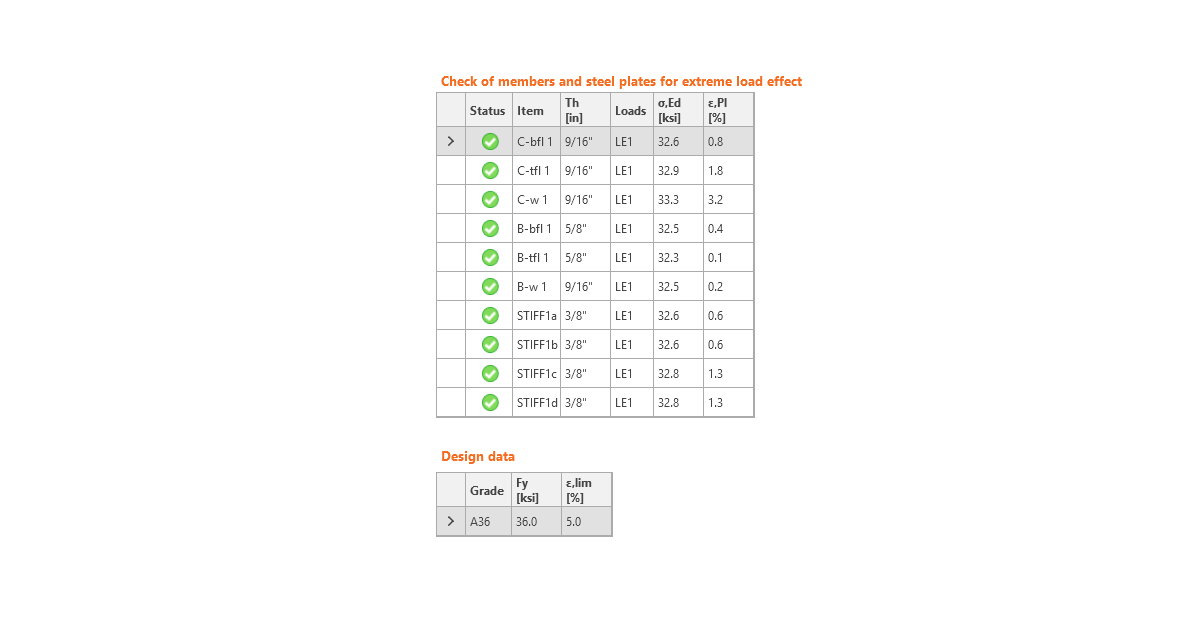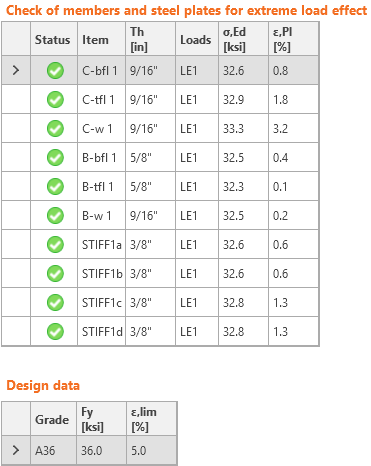### Choose language# Code-check of steel plates (AISC)

$$The resulting equivalent stress (HMH, von Mises) and plastic strain are calculated on plates. When the yield strength (in LRFD multiplied by material resistance factor ϕ = 0.9, in ASD divided by material safety factor Ω = 1.67, which are editable in Code setup) on the bilinear material diagram is reached, the check of the equivalent plastic strain is performed. The limit value of 5 % is suggested in Eurocode (EN1993-1-5 App. C, Par. C8, Note 1). This value can be modified in the Code setup, but verification studies were made for this recommended value.The plate element is divided into five layers, and elastic/plastic behavior is investigated in each of them. The program shows the worst result of all of them.The CBFEM method can provide stress a little bit higher than yield strength. The reason is the slight inclination of the plastic branch of the stress-strain diagram, which is used in the analysis to improve the stability of the interaction calculation. This is not a problem for practical design. The equivalent plastic strain is exceeded at higher stress, and the joint does not satisfy anyway.$$